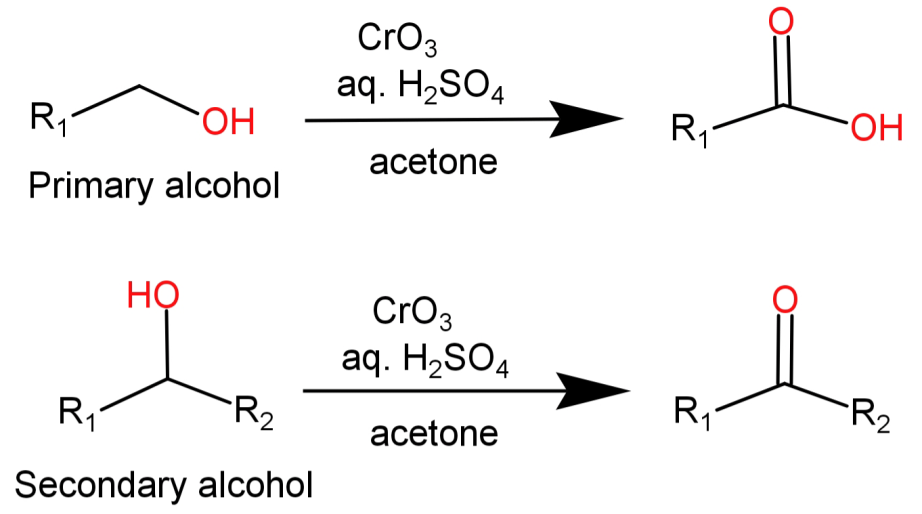# Jone’s reagent is:A.Acidified ${K_2}C{r_2}{O_7}$ solutionB. Alkaline ${K_2}C{r_2}{O_7}$ solutionC.$Cr{O_3}$ in aqueous acetoneD. A solution of ${K_2}C{r_2}{O_7}$/ ${H_2}S{O_4}$ in aqueous ethanolVerified
203.7k+ views
Hint:Jone’s reagent is a strong oxidising agent which oxidises primary and secondary alcohols to carboxylic acids and aldehydes respectively. This reagent is acidic in nature.

-Jone’s reagent is basically a solution of chromium trioxide ($Cr{O_3}$) in dilute sulphuric acid (dil. ${H_2}S{O_4}$). Alternatively sodium dichromate ($N{a_2}C{r_2}{O_7}$) or potassium dichromate (${K_2}C{r_2}{O_7}$) can also be used in place of chromium trioxide. This acidic mixture is added to the acetone solution of the substrate to move forward with the reaction.
So, we can say that Jone’s reagent is a mixture of chromic trioxide and dilute sulphuric acid ($Cr{O_3} + {H_2}S{O_4} + {H_2}O$).
It is an oxidising reagent and the reaction it is involved in is known as Jone’s oxidation reaction.
-It causes the oxidation of primary alcohols to carboxylic acids and that of secondary alcohols to ketones respectively.-Since this reagent is acidic in nature the substrate present in acetone is essentially titrated by this oxidant solution.
This oxidation reaction begins with the reaction of $Cr{O_3}$ with the acid ${H_2}S{O_4}$ which forms chromic acid or dichromic acid (in solutions which are more concentrated). Then the primary or secondary alcohol reacts with chromic acid and gets oxidised while chromic acid gets reduced in the process.
-This type of oxidation is very rapid and exothermic. Since it is a very strong oxidising agent it can also reduce aldehydes to carboxylic acids.

So, the correct option is: (C) $Cr{O_3}$ in aqueous acetone.

Note:
The presence of sulphuric acid may result in some side reactions as well so to minimise them its amount can be minimised. But remember that with decrease in its concentration the oxidising power of Jone’s reagent also decreases.